IF YOU CAN SEE THIS go to /sfa/Nuprl/Shared/Xindentation_hack_doc.html
NumThyExamples
Nuprl Section: NumThyExamples - Number Theory Examples
Here are several examples of proofs about numbers. Each consists of a formal proof and an informal gloss of it.

Each is an improvement (in this author's opinion - sfa ) of an extant proof; the original proofs were chosen by Amanda Holland-Minckley for use in a study on the natural language rendering of Nuprl proofs. Of course, whatever defects may be borne by the originals are no reflection upon that study. This was simply an opportunity to reexamine these proofs for readability; indeed, the "glossability" of the formal proof is one useful criterion for readability.

Here are the new proofs (the old ones are linked with "[old]").

Thm*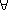a: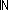b: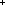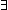q:r:ba = q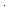b+r [old]

Thm*n:. CoPrime(fib(n),fib(n+1)) [old]

Thm*b:a: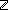u,v:. GCD(a;b;ua+vb) [old]

Thm*i:{8...}.m,n:. 3m+5n = i [the "stamps" problem; old version unavailable]